# How to Visualize Proportions in R

There are many ways to show parts of a whole. Here are quick one-liners for the more common ones.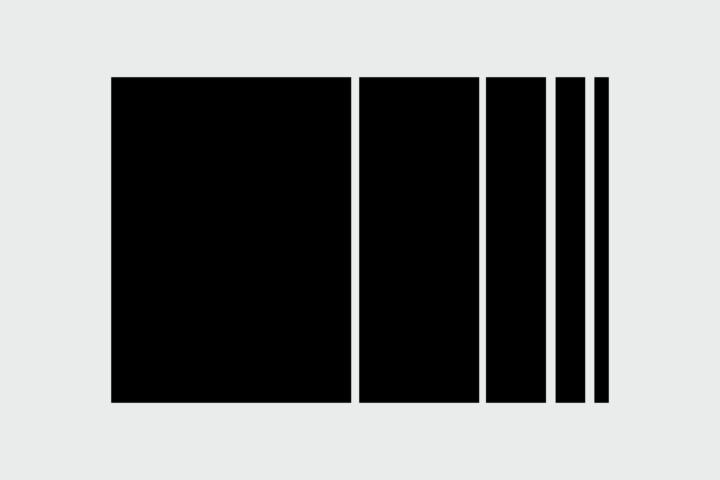You can visualize proportions in a lot of ways. However, there are visualization types that are commonly used, which typically means they’re more commonly understood by a lot of people.

In this tutorial, we quickly go through what you can use in R, focusing on the types that maintain the parts-of-a-whole metaphor. I won’t harp on whether a method is useful or not. Instead, I’ll give you the tools, and you can decide what you want to do with it.

### Before Getting Started

This is an R tutorial, so you should have R installed to work through the examples. Here’s a short guide here if you don’t have it yet.

You also need some data. With the R console open, enter the below for some numbers to work with.

```# Some data
pct <- c(10, 20, 30, 40)
shades <- c("white", "lightgray", "darkgray", "black")
categories <- c("a", "b", "c", "d")
```

And that’s it. You use one package, but you can install that when the example comes.

### Pie Chart

How can you not start with this one? Base R provides `pie()` to make everyone’s favorite proportional chart. Pass a vector of values, and the function does the rest. If you pass raw counts, the function does the math for percentages. Optionally, you can specify label names with the `labels` parameter and color with `col`. The order of these vectors should correspond to the values vector.

```pie(pct, labels = categories, col = shades)
```

As expected, here’s your pie chart in all its circular glory.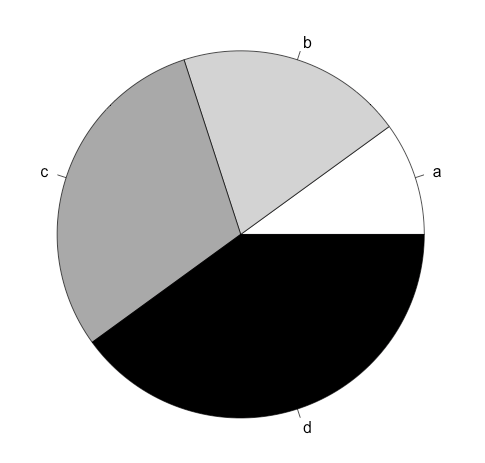### Donut Chart

R doesn’t provide a donut chart function out of the box, but you can quickly make one by modifying a pie chart. Just slap a circle in the middle using `symbols()`.

```pie(pct, labels = categories, col = shades)
symbols(0, 0, circles = 1, add=TRUE, bg="white")
```

The first line with `pie()` is the same as the previous example. As for `symbols()`, you pass the x- and y-coordinate, which is (0, 0) in this case, set the circle radius to 1, add to the existing pie, and set the color to white.

Donut?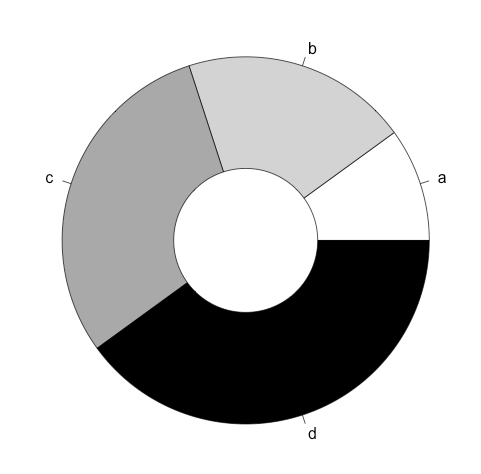Sorry, I couldn’t resist.

### Square Pie Chart

We covered these in a previous tutorial. Again, there’s no function from base R, but we can use the custom function from said tutorial for a `squarePie()` function.

To change your working directory: In RStudio, go to the Session → Set Working Directory menu. In standard R, go to Misc → Change Working Directory.Before you use `source()` to load square-pie-chart.R, make sure your current working directory in R is set to where you downloaded this tutorial’s code.

The `squarePie()` function takes a vector of values and a corresponding vector of colors.

```source("lib/square-pie-chart.R")
```

Square: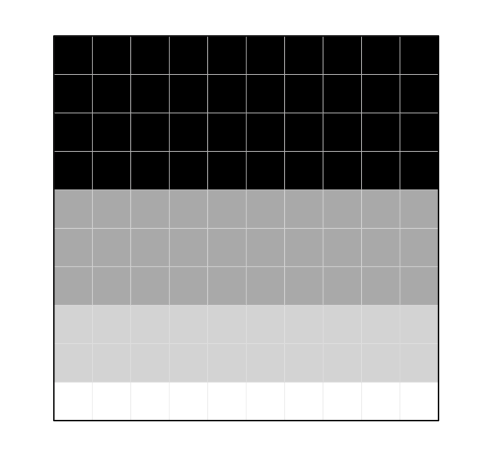For more on square pie charts, check out the tutorial.

### Area Graph

The area graph can be useful to show proportions over time. Like the square pie chart, we covered these in a previous tutorial, and the end result was a function we can reuse.

First, load the code with `source()`. Again, your working directory must be set to this tutorial’s download folder.

```source("lib/areagraph.R")
```

Then we create a data frame where each row represents a layer of the area graph. Each column represents a segment of time.

```pct_over_time <- rbind(pct1=pct, pct2=2*pct)
pct_df <- data.frame(pct_over_time)
```

Plug the data frame into `areaGraph()`.

```areaGraph(pct_df, type=0)
```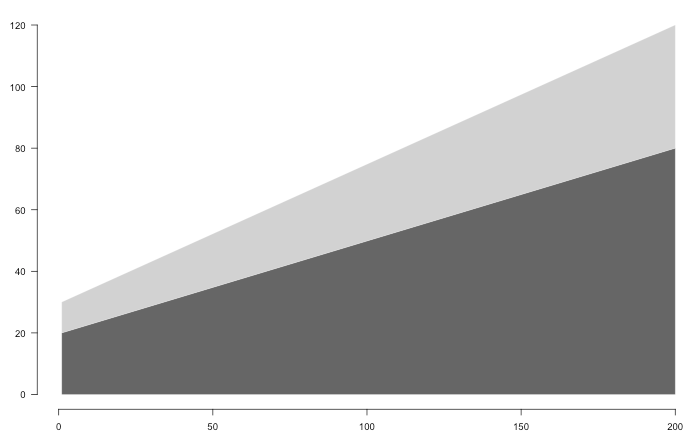Try setting `type` to 1 or 2 for different baseline offsets.

For more on area charts, check out the tutorial.

### Stacked Bar Chart

You can view the contents of any data structure in R by entering the variable name. For example, enter “pct_over_time” to see what the matrix looks like.The stacked bar chart is the stacked area chart’s discrete cousin. The `barplot()` function in R will take care of it for you, but instead of the data frame used with `areaGraph()`, you provide a matrix.

```barplot(pct_over_time)
```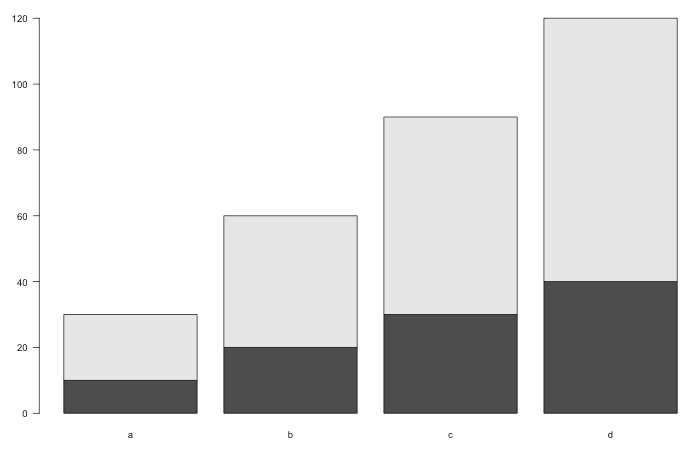### Treemap

This one relies on a package, aptly named treemap. Use `install.packages()` to install, as shown below. Then load the package with `library()`.

```install.packages("treemap", dependencies = TRUE)
library(treemap)
```

The function takes a data frame of values, categories, and colors as its columns. So each row of the data frame represents a value, what color and/or size the rectangle in the treemap should be, and what it should be labeled.

```vals <- data.frame(pct=pct, cat=categories, col=shades)
```

Enter `?treemap` to see more options.Pass the newly constructed data frame to `treemap()`, and then use the column names to specify what makes what. In this example, the `index` (i.e. the categories) is the “cat” column, the size of the rectangle (`vSize` parameter) is based on “pct”, color (`vColor`) is set by “col”, and set `type` to “color” to specify that colors are already defined.

```treemap(vals, index="cat", vSize="pct", vColor="col", type="color")
```

Treemaps are actually more useful for hierarchical data, but this kind of works too.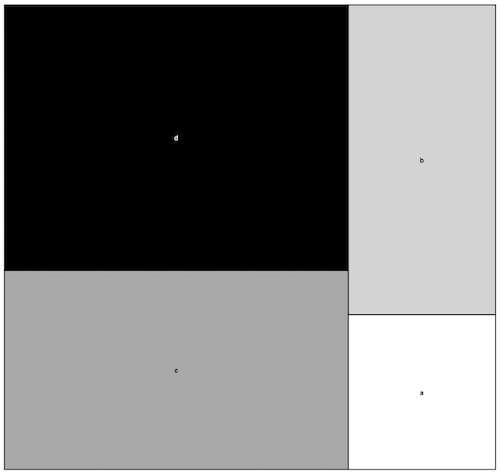### Wrapping Up

That should cover about 90 percent of your proportion problems easily. Of course, there’s no rule that you must stick to the parts-of-a-whole metaphor. So if you’re willing to get away from that, you can use other stand-bys like a bar chart or a dot plot.

Become a member to support an independent site and learn to make great charts.